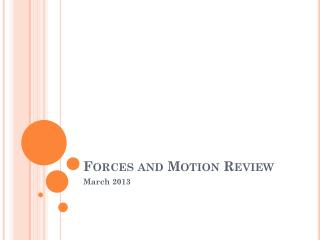DownloadDownload PresentationForces and Motion Review

# Forces and Motion Review

Télécharger la présentation## Forces and Motion Review

- - - - - - - - - - - - - - - - - - - - - - - - - - - E N D - - - - - - - - - - - - - - - - - - - - - - - - - - -
##### Presentation Transcript

1. Forces and Motion Review March 2013

2. Forces & Work • Work is done when…. a force moves an object. • If you push a rock and it doesn’t move, has work been done? • What is a force? • Explain what happens when the cue ball hit another ball in pool table. Which one of Newton’s laws shows this example? • http://www.youtube.com/watch?v=NWE_aGqfUDs

3. Motion • When the smaller gear begins to turn that causes the larger gear to turn. • Therefore, when the smaller gear makes one full turn, the larger gear would have made a partial turn.

4. Comparing the Stretch of the rubber band Lab What happened to the rubber band when we stretched it? What happened when we added pencils? Why was the stretch less on the rubber band when the pencils were used?

5. Line Plot • How could you create a line plot with the data we have from the rubber band lab?

6. Friction • How does friction affect movement? • Friction can slow, stop or even prevent movement. • Earlier in this unit, we discussed how friction affects your feet when you slip on a wet floor. • How is friction involved?

7. Forces The greater the net force.. The force that keeps a satellite in orbit… • The greater the acceleration • Can you explain why? • Gravity! • What other objects in space does gravity affect?

8. Non-examples of forces Examples Non-examples • Magnets • Apple falling • Picking up a book • A tree standing in the middle of a corn field • What are other non-examples?

9. Unbalanced forces • What is the definition of an unbalanced force? • Hint: Look in chapter 11 or the glossary! • Example: In a tug of war game, the rope is pulled in the direction of the greatest force.

10. Forces Electricity and Magnetism Application: Roller Coasters • How do they act on different objects? • Answer: They can either push or pull objects. • What makes your body rise out of its seat at the top of a hill as you are riding the roller coaster? • Inertia, gravity, electricity or friction?

11. Simple Machines Can you name all the simple machines?

12. Simple Machines • Wheel and Axle • Two examples are: • Door knob • Bicycle wheel • Wedge • A few examples are: • Teeth biting into an apple • An ax splitting wood • A doorstop holding a door open

13. Simple & Complex Machines • Lever • An example of a lever is: • A screwdriver being used to pry the lid off a paint can • Complex machine • Composed of two or more simple machines • Example: Bicycle • A bicycle uses mainly human force to operate the simple machines in it